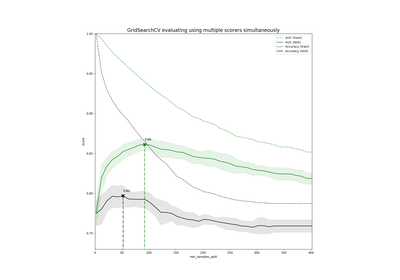# `sklearn.metrics`.make_scorer¶

`sklearn.metrics.``make_scorer`(score_func, greater_is_better=True, needs_proba=False, needs_threshold=False, **kwargs)[source]

Make a scorer from a performance metric or loss function.

This factory function wraps scoring functions for use in GridSearchCV and cross_val_score. It takes a score function, such as `accuracy_score`, `mean_squared_error`, `adjusted_rand_index` or `average_precision` and returns a callable that scores an estimator’s output.

Read more in the User Guide.

Parameters: score_func : callable, Score function (or loss function) with signature `score_func(y, y_pred, **kwargs)`. greater_is_better : boolean, default=True Whether score_func is a score function (default), meaning high is good, or a loss function, meaning low is good. In the latter case, the scorer object will sign-flip the outcome of the score_func. needs_proba : boolean, default=False Whether score_func requires predict_proba to get probability estimates out of a classifier. needs_threshold : boolean, default=False Whether score_func takes a continuous decision certainty. This only works for binary classification using estimators that have either a decision_function or predict_proba method. For example `average_precision` or the area under the roc curve can not be computed using discrete predictions alone. **kwargs : additional arguments Additional parameters to be passed to score_func. scorer : callable Callable object that returns a scalar score; greater is better.

Examples

```>>> from sklearn.metrics import fbeta_score, make_scorer
>>> ftwo_scorer = make_scorer(fbeta_score, beta=2)
>>> ftwo_scorer
make_scorer(fbeta_score, beta=2)
>>> from sklearn.model_selection import GridSearchCV
>>> from sklearn.svm import LinearSVC
>>> grid = GridSearchCV(LinearSVC(), param_grid={'C': [1, 10]},
...                     scoring=ftwo_scorer)
```

## Examples using `sklearn.metrics.make_scorer`¶Demonstration of multi-metric evaluation on cross_val_score and GridSearchCV